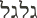## Godly Circle Diameter 66/ Circumference 207

Godly Circle Diameter 66/ Circumference 207.

CircleA circle (black), which is measured by its circumference (C), diameter (D) in cyan, and radius (R) in red; its centre (O) is in magenta.

circle is a simple closed shape. It is the set of all points in a plane that are at a given distance from a given point, the centre.  It is the curve traced out by a point that moves so that its distance from a given point is constant.

How the ancient four elements structure the circle: Four elements were known in antiquity.  They were recognized through the Middle Ages:  (1) Earth (2) air (3)  fire and (4) water.  In the 21st century, we laugh at this. After all, we know there are 92 re-generative and re-occurring elements. But in the process, we have overlooked a lost and long fogtteen code: The 4 basic elements of organic chemistry are carbon, hydrogen, nitrogen and oxygen. These are the actual elements behind the enigmatic, alchemical four just listed.

• The source of fire on stars is hydrogen, atomic number-1
• Our air is comprised of mostly nitrogen, atomic number-7.  Nitrogen accounts for 78% of the atmosphere, oxygen is second in size with 21%.
• The hardest earth substance is diamond. It is consists of compressed carbon.  Carbon’s atomic number is -6.
• By atomic weight, our water is mostly oxygen Water’s formula is H2O. One atom of  oxygen has 8 protons and 8 neutrons. Two hydrogen atoms only have 2 protons, i.e. one per atom. An atom of oxygen is approximately 16 times heavier than an atom of hydrogen.

The atomic number total of the chemical four elements is:  1 + 6 + 7 + 8 = 22. Twenty-two, by the four elements,  goes around the zodiac three times. Thus, 3 x 22 = 66.

### Godly Circle on the Tree of Life

The three tenses of time in Hebrew equate with 66. This is by the ancient rules of gematria. Look this term up on Wikipedia.

(1) “Was” (past)  It is spelled with Hebrew letters (hei-yud-hei) with a gematria ( numerical equivalency) of 20;

(2) “Am” (present)  (hei-vav-hei) with a gematria of 16.

(3) ” Will be” (future)  (yud-hei-yud-hei) with a gematria of 30.

The total of the three tenses becomes 20 +16+ 30 = 66. We have just seen how both the “elements” and time are equated with 66. Space and time, in this manner, equate with #66.

### Godly Circle of 66 Diameter 207 Circumference  on theTree of Life

• The Hebraic parallel is The Tree of Life. It has 10 emanations that are depicted as circles. As mentioned, at one time letters and numbers shared the same symbol, The Hebrew word for circle( (galgal)  equals 66.  Hebrew  is written right to left. Gimmel (1st letter) = 3;  lamed (second letter)= 30.  Since these letters are repeated (as pictured) in the Hebrew word, galgal, 2 x 33 =66.